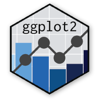Show Sidebar Hide Sidebar# geom_quantile in ggplot2

How to use geom_quantile with Plotly.

### New to Plotly?

Plotly's R library is free and open source!
You can set up Plotly to work in online or offline mode.
We also have a quick-reference cheatsheet (new!) to help you get started!

### Version Check

Version 4 of Plotly's R package is now available!
Check out this post for more information on breaking changes and new features available in this version.

library(plotly)
packageVersion('plotly')

##  '4.9.1'


### Basic Example

While common linear regression is a method of estimating the conditional mean of variable y based on the values of variable(s) x, quantile regression is a method that can give the conditional median (50th percentile) as well as any other quantile.

This dataset gives the effect of the mother's weight on her baby's birth weight, further divided according to whether the mother smokes or not. The line shows the median birth weight conditional on these two other variables.

library(plotly)
library(MASS)
library(quantreg)

df <- MASS::birthwt

df <- with(df, { #Make sure variables properly show up as categories
race <- factor(race, labels = c("white", "black", "other"))
ptd <- factor(ptl > 0)
ftv <- factor(ftv)
levels(ftv)[-(1:2)] <- "2+"
data.frame(low = factor(low), age, lwt, race, smoke = (smoke > 0),
ptd, ht = (ht > 0), ui = (ui > 0), ftv, bwt)
})

p <- ggplot(df, aes(lwt, bwt, colour = smoke)) +
geom_point(size = 1) +
geom_quantile(quantiles = 0.5)

p <- ggplotly(p)

p


### With Quantiles

geom_quantile is capable of showing more than just the conditional median: here we show the median, the 10th percentile, and 90th percentiles as well. We see that, among nonsmokers, the likelihood of underweight babies decreases significantly as the mother's weight increases, but that mothers of all weights are roughly equally likely to give birth to the heaviest babies. Conversely, among smoking mothers, the likelihood of underweight babies seem to increase as mother's weight increases.

Given the small sample size for this dataset, it's wise not to draw too many conclusions; this is meant to illustrate the purpose of quantile regression. You can also adjust the lines' appearance.

library(plotly)
library(MASS)
library(dplyr)

df <- MASS::birthwt

df <- with(df, {
race <- factor(race, labels = c("white", "black", "other"))
ptd <- factor(ptl > 0)
ftv <- factor(ftv)
levels(ftv)[-(1:2)] <- "2+"
data.frame(low = factor(low), age, lwt, race, smoke = (smoke > 0),
ptd, ht = (ht > 0), ui = (ui > 0), ftv, bwt)
})

p <- ggplot(df, aes(lwt, bwt, colour=smoke)) +
geom_point(size = 1) +
geom_quantile(quantiles = c(0.1, 0.5, 0.9), size = 2, aes(alpha = ..quantile..)) +
scale_alpha(range = c(0.3, 0.7))
p <- ggplotly(p)

p


Reference: ggplot2 docs

### Reference